# What to Do If: Bookworm Makes You Feel StupidPopCap’s Bookworm is a fantastic franchise. I’ve played a lot of Bookworm in my day, particularly because my company’s first game, years and years ago, was a spelling word game.  But I never became very good at word games, despite playing dozens of them while surveying the genre’s design landscape.

#### Word Game Players

Other people are insanely good at word games.  We spent a few days at the Independent Games Festival in 2004 demoing Beesly’s Buzzwords, and the occasional word game wizard would come forth from the crowd to spell 7- and 8- letter words with alarming rapidity.  I’ve also witnessed people who play word games daily but never seem to get any better, but still seem to enjoy the experience.

I don’t get any better.  And Bookworm is now available on the iPhone–at the everything-on-the-iPhone-is-cheap-as-dirt price of \$0.99–so I thought I’d check it out.  It’s the usual PopCap fare, with fantastic polish, some new features, solid production…but it still makes me feel dumb.

#### How to Play Spelling Games

The skill of a word game is “chunking” your mental searches into abstract-but-useful combinations of letters.  They’re hard to see at first because we rarely build mental associations with them.  The beginner searches for consonant-vowel-consonant patterns:  pot, dog, cat, tip, etc.  However, most spelling games begin to punish short words, and Bookworm is no exception.  To expand their search the intermediate player begins to see prefixes and suffixes, like “ing” or “ers”.  More advanced players see all kinds of subtle pairs, like “av”, “dg”, “ch”, “dr”, “gr” (think changing, dodge, greater, avalanche).

#### Automating Bookworm

I get all of this, but I still have a hard time increasing my mental lookup times for bizarre letter combinations.  I don’t like the feeling of floundering at a task that I know is more well-suited to a computer.  So I thought:  Why not have a computer do the actual work?  I saw a great article on HN about a guy who wrote an automated Sudoku solver, where you take photos of printed puzzles and it solves them.  Doing the same with Bookworm should be much easier, since I can screenshot the game and deal with pixel-perfect data.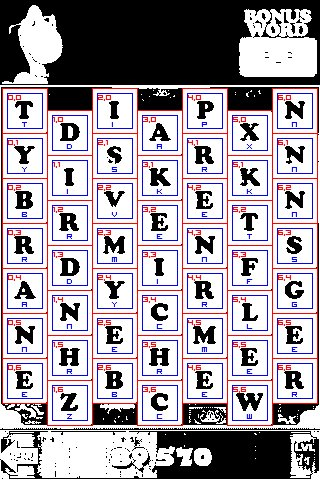And all of the words for this board:

8-Letter Words:
Briskets B(0,2) R(1,2) I(1,1) S(2,1) K(3,1) E(4,2) T(5,2) S(6,3)
Sirvente S(2,1) I(1,1) R(1,2) V(2,2) E(3,2) N(4,3) T(5,2) E(4,2)

7-Letter Words:
Branner B(0,2) R(0,3) A(0,4) N(0,5) N(1,4) E(2,5) R(1,5)
Birdmen B(0,2) I(1,1) R(1,2) D(1,3) M(2,3) E(3,2) N(4,3)
Brisker B(0,2) R(1,2) I(1,1) S(2,1) K(3,1) E(4,2) R(4,1)
Brisket B(0,2) R(1,2) I(1,1) S(2,1) K(3,1) E(4,2) T(5,2)
Reynard R(1,5) E(2,5) Y(2,4) N(1,4) A(0,4) R(0,3) D(1,3)
Becrime B(2,6) E(2,5) C(3,4) R(4,4) I(3,3) M(2,3) E(3,2)
Reynard R(3,5) E(2,5) Y(2,4) N(1,4) A(0,4) R(0,3) D(1,3)
Parents P(4,0) A(3,0) R(4,1) E(4,2) N(4,3) T(5,2) S(6,3)

6-Letter Words:
Branny B(0,2) R(0,3) A(0,4) N(0,5) N(1,4) Y(2,4)
Brandy B(0,2) R(0,3) A(0,4) N(1,4) D(1,3) Y(2,4)
Arrive A(0,4) R(0,3) R(1,2) I(1,1) V(2,2) E(3,2)
Risker R(1,2) I(1,1) S(2,1) K(3,1) E(4,2) R(4,1)
Driven D(1,3) R(1,2) I(1,1) V(2,2) E(3,2) N(4,3)
Sirdar S(2,1) I(1,1) R(1,2) D(1,3) A(0,4) R(0,3)
Skeets S(2,1) K(3,1) E(3,2) E(4,2) T(5,2) S(6,3)
Veiner V(2,2) E(3,2) I(3,3) N(4,3) E(4,2) R(4,1)
Venter V(2,2) E(3,2) N(4,3) T(5,2) E(4,2) R(4,1)
Minter M(2,3) I(3,3) N(4,3) T(5,2) E(4,2) R(4,1)
Brewer B(2,6) R(3,5) E(4,6) W(5,6) E(5,5) R(6,6)
Entera E(3,2) N(4,3) T(5,2) E(4,2) R(4,1) A(3,0)
Crewel C(3,4) R(3,5) E(4,6) W(5,6) E(5,5) L(5,4)
Cymene C(3,4) Y(2,4) M(2,3) E(3,2) N(4,3) E(4,2)
Reeler R(3,5) E(4,6) E(5,5) L(5,4) E(6,5) R(6,6)
Crewel C(3,6) R(3,5) E(4,6) W(5,6) E(5,5) L(5,4)
Parent P(4,0) A(3,0) R(4,1) E(4,2) N(4,3) T(5,2)
Mercer M(4,5) E(4,6) R(3,5) C(3,4) E(2,5) R(1,5)
Kraken K(5,1) R(4,1) A(3,0) K(3,1) E(3,2) N(4,3)
Kraken K(5,1) R(4,1) A(3,0) K(3,1) E(4,2) N(4,3)
Terais T(5,2) E(4,2) R(4,1) A(3,0) I(2,0) S(2,1)
Steeks S(6,3) T(5,2) E(4,2) E(3,2) K(3,1) S(2,1)

#### Processing Bookworm

The first step is to get data out of Bookworm itself.  The iPhone provides a screenshot function–press home and power simultaneously–and you can email the resulting JPG to yourself from Camera Roll (you can also pull it out of Xcode Organizer if you’re a developer).  Bookworm saves your board when you exit, so it’ll be waiting upon your return.  This gives you a nice clean JPG: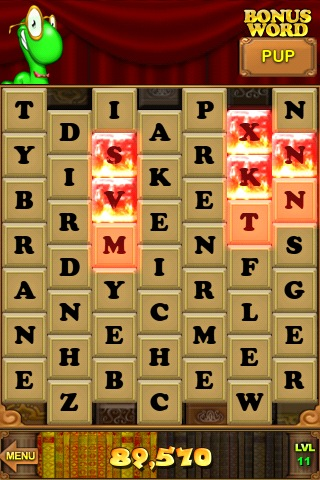Next, you’re going to want to clean up some of the data.  This is especially important for boards where you have flaming tiles going on. The simplest solution is to threshold the pixels.  I used the fantastic ImageMagick suite of command-line image tools for this, with:  convert -threshold 25% input.jpg output.png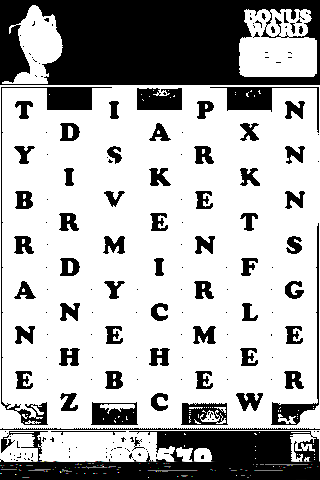From there it’s a simple matter of identifying the letters.  You can’t rely on pixel-perfect matches, due to the flaming tiles and other effects, but ImageMagick provides a handy compare tool in their suite.  I’m using: compare -metric mae thisTile.png letters/someLetter.png difference.png 2> compare.txt (for whatever reason ImageMagick on OSX outputs the numerical analysis to standard error, so I have to pipe it to get it out).  It even gives you a nice display of the difference between the two files: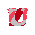You do need to capture each individual letter, but this is pretty easy to do, since you’re already isolating each letter to compare it against all known letters.  It took two minutes to go in and rename the temporary grid output to build my identification library: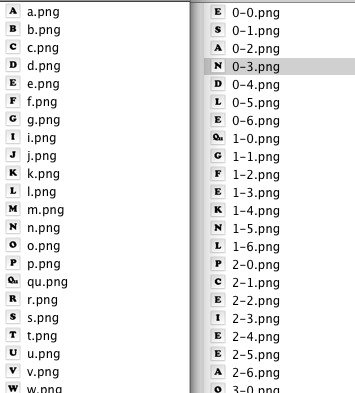Edit: Looks like I forgot to save an ‘H’ when I did the letter training. In the above images they’re matching as ‘R’, which is the next closest visual match. Whoops!

From there it’s a simple matter of recursing the entire board, looking for matches.  I don’t do anything particular clever on the search (it’s actually the fastest part of the process at just a few seconds).  I am checking for premature search death.  For instance, if the first few letters of a match are xyt, you can kill off the entire tree instead of continuing to search for words.  My enable2k dictionary of 180k words results in 380k possibilities (“strawberry” is stored as “str”, “stra”, “straw”, etc).

#### What’s Next?

I did this whole thing as one big experiment.  My thought process went from “man, I feel like a shitty computer when play word games–a computer could easily do this” to “oh yeah?  prove it, talky talk-man”.  The three hours that it took to make this were certainly more fun for me than three hours of actual Bookworm play would have been.  That was just my goal all along:  To find some way to turn a frustrating experience into a fun one.

I may launch it as a service, actually.  It would be amusing to unleash this on the hardcore Bookworm fans.  You would take a screenshot, email it to some address, and get your best words emailed back in a minute or two.  I wonder if people would actually use that?  Only one way to find out…

Bookworm and the Lex character are registered trademarks of PopCap Games.  For the record, Bookworm for the iPhone has no online scoring.  I can’t do anything with this except feel less retarded when I play.

P.P.S. Wurdle

I did Wurdle, too. It uses an 8-neighbor grid, so it actually has more words (and the max grid size is 8×8 compared to Bookworm’s 7×7). The average 8×8 Wurdle board has ~1500 possible word solutions. Word lengths over 9 are rare and seem to appear in 25% or fewer layouts. Have a look: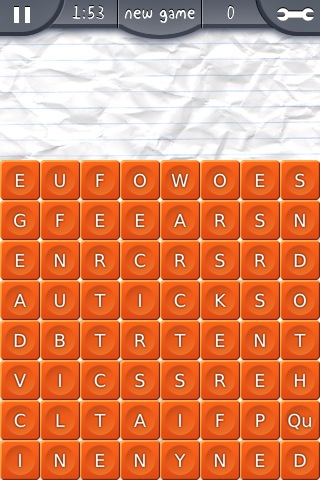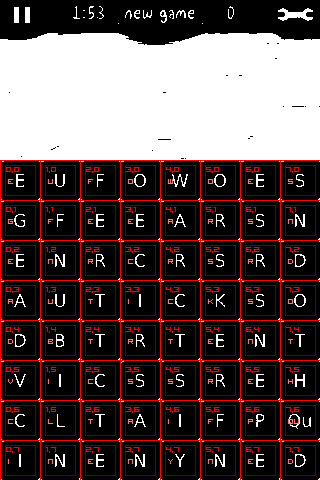Words:

12-Letter Words:
Criticasters C(3,2) R(4,2) I(3,3) T(2,4) I(1,5) C(2,5) A(3,6) S(3,5) T(4,4) E(5,4) R(5,5) S(4,5)

11-Letter Words:
Criticaster C(3,2) R(4,2) I(3,3) T(2,4) I(1,5) C(2,5) A(3,6) S(3,5) T(4,4) E(5,4) R(5,5)

9-Letter Words:
Freewares F(1,1) R(2,2) E(2,1) E(3,1) W(4,0) A(4,1) R(5,1) E(6,0) S(6,1)
Fricassee F(1,1) R(2,2) I(3,3) C(3,2) A(4,1) S(5,2) S(6,3) E(5,4) E(6,5)
Neuritics N(1,2) E(0,2) U(1,3) R(2,2) I(3,3) T(2,4) I(1,5) C(2,5) S(3,5)
Reasserts R(2,2) E(3,1) A(4,1) S(5,2) S(6,3) E(5,4) R(5,5) T(4,4) S(4,5)
Rectrices R(2,2) E(3,1) C(3,2) T(2,3) R(3,4) I(3,3) C(4,3) E(5,4) S(6,3)
Crassness C(3,2) R(4,2) A(4,1) S(5,2) S(6,3) N(6,4) E(5,4) S(4,5) S(3,5)
Reasserts R(4,2) E(3,1) A(4,1) S(5,2) S(6,3) E(5,4) R(5,5) T(4,4) S(4,5)
Rectrices R(4,2) E(3,1) C(3,2) T(2,3) R(2,2) I(3,3) C(4,3) E(5,4) S(6,3)
Crassness C(4,3) R(4,2) A(4,1) S(5,2) S(6,3) N(6,4) E(5,4) S(4,5) S(3,5)
Stricture S(4,5) T(4,4) R(3,4) I(3,3) C(3,2) T(2,3) U(1,3) R(2,2) E(2,1)
Frenetics F(5,6) R(5,5) E(6,5) N(6,4) E(5,4) T(4,4) I(3,3) C(4,3) S(5,2)

8-Letter Words:
Efferent E(0,0) F(1,1) F(2,0) E(3,1) R(2,2) E(2,1) N(1,2) T(2,3)
Generics G(0,1) E(0,2) N(1,2) E(2,1) R(2,2) I(3,3) C(4,3) S(5,2)
Gentrice G(0,1) E(0,2) N(1,2) T(2,3) R(2,2) I(3,3) C(3,2) E(3,1)
Efferent E(0,2) F(1,1) F(2,0) E(3,1) R(2,2) E(2,1) N(1,2) T(2,3)
Enticers E(0,2) N(1,2) T(2,3) I(3,3) C(3,2) E(3,1) R(4,2) S(5,2)
Dicastic D(0,4) I(1,5) C(2,5) A(3,6) S(3,5) T(4,4) I(3,3) C(3,2)

1.PogoFan

Cheat program developers have been making bank for many years by provide these tools for games on Pogo.com.

2.Matthew

Oh yeah–I’m under no illusions that any competitive multiplayer environment isn’t going to have rampant cheating like this (and I’m sure physical prizes available from Pogo contribute to it, although my guess is people would do it for the notoriety alone). Online poker is even more fascinating, as many cheat bots connect with each other to increase their visibility into the deck, with multiple machines in the same game sharing their card counts.

This was just my way of enjoying Bookworm–the 3 hours I spent creating this were far more enjoyable for me than three hours of actual Bookworm playing would have been.

I’ve also wanted to create a computer vision/mechanical solution to play Rock Band, although that’s a little bit bigger than a lazy Sunday project…

3.Matthew

The band leader bot would need to squawk out “star power!” when it detected a filled meter, and the other bots would need microphones and the ability to separate the leader bot’s commands from the background music. Clearly.

4.bud

You should make an iPhone app that lets you send screen shots from Wurdle and Bookworm to your server for cheating. People would drop 99 cents on that easy.

I actually think over 50% of any electronic gaming market is the ‘cheat’ market.

### Webmentions

• Word play: beating PopCap’s Bookworm with science | VENUS PATROL August 4, 2009

[…] is still active for a few more days [App Store link]), Flashbang head Matthew Wegner drops this: an automated OCR program to beat the ‘worm at his own game: I still have a hard time increasing my mental lookup times for bizarre letter combinations. I […]